# Number the Visible Rows in Excel AutoFilter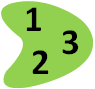When you use an Excel AutoFilter to filter a list, usually the count of visible records appears in the Status Bar, at the bottom left of the Excel window. (Note: If the Status Bar shows Filter Mode, instead of the record count, you can use one of the workarounds shown here – Status Bar shows Filter Mode.)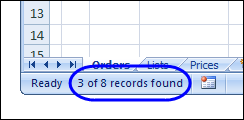That's helpful if you're looking at the results on the screen, but not much help if you print the filtered list and give it to someone else. To make things easier for them, you can add record numbers that will print for each row.
For example, in the screenshot below, the visible rows are 2, 4, 5 and 6, and the numbers in column B are 1, 2, 3 and 4.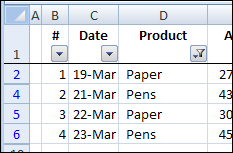### Add Record Numbers to a Filtered List

In column B of our list, if we just type the numbers, or use a simple formula, such as =B2+1 the numbers won't change if the list is filtered. In the screenshot below, the second record is hidden by the filter, and the record count shows as 1, 3, 4, 5.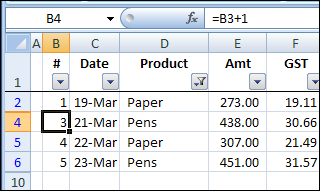Instead of using the simple formula shown above, if you want to show the record numbers for the visible records, you can use the SUBTOTAL function. It is designed to ignore rows that are hidden by a filter, so its result will change if rows in our list are hidden.
WARNING: Don't use this technique if you plan to use Excel's Subtotal feature (Data>Subtotals) -- it may delete your table when you remove the Subtotals.
In our list, there will always be a date in column C, so we can use the SUBTOTAL function to count the visible dates.
=SUBTOTAL(2, C\$1:C2)
We're counting dates, so the first argument, 2, tells Excel to count the numbers in the range. If you want to count text entries instead, use (COUNTA) as the first argument.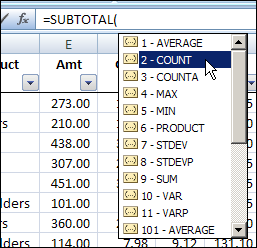The second argument, C\$1:C2 is the range of cells that we want to count.
• The first cell, C\$1, has an absolute row reference, so when we copy the formula down, that part of the formula won't change. We always want the count to start in row 1.
• The second cell, C2, has a relative row reference, so when we copy the formula down, that part of the formula WILL change. We want the count to end at the row the formula is in.
Now, when you apply an Excel AutoFilter, or show all the records, your record numbers in column B will change.

### Problems Hiding the Last Filtered Row

The SUBTOTAL function works well, and renumbers our rows as expected, but there's something wrong. In the screenshot below, the list is filtered for the products Paper or Staplers, as you can see in the AutoFilter tooltip. Can you spot the problem?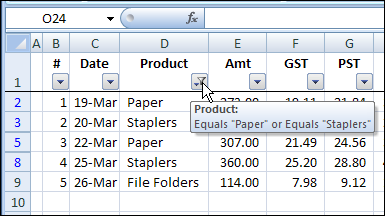Although the list is filtered for Paper or Staplers, the File Folders record in row 9 is also visible. Also, its row number isn't blue, like the row numbers for rows 2, 3, 5 and 8.
When you use an AutoFilter, Excel creates a hidden named range for the database. Using Jan Karel Pieterse's Name Manager utility, I can see the definition for Orders!_FilterDatabase. Even though the list ends in row 9, the named range stops at row 8:  =Orders!\$B\$1:\$H\$8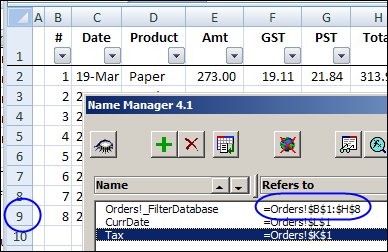If there's a SUBTOTAL function in the last row of that database, Excel decides that it's a special row for the list's totals, and it's not included in the named range.
So, if you want your last row hidden when using the SUBTOTAL function in a filtered list, you can use one of the following workarounds.

#### Workaround 1: Add a Dummy Row

Usually, I add a dummy row at the end of the list, with just the SUBTOTAL function, or other dummy data. Then, that row is treated as the last row, and all the real data is shown or hidden, based on the AutoFilter criteria.
In the screenshot below, the SUBTOTAL function is copied down to row 10, and when the AutoFilter is applied, row 9 is hidden. There's no date in row 10, so it doesn't affect the record numbering.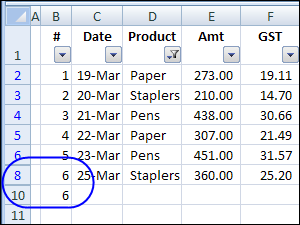### Workaround 2: Change the SUBTOTAL formula

Today I found another workaround, that's much better than the dummy row solution. Dick Kusleika wassubtotalling filtered rows, and discovered that he could fix the problem by typing two minus signs in front of the SUBTOTAL function. (I left a space between the minus signs below, just for clarity. The formula will work with or without the space.)
=- -SUBTOTAL(2, C\$1:C2)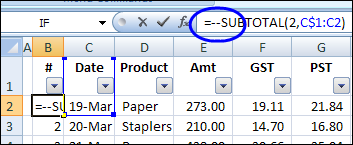With Dick's solution, there's no need for an extra row, so the worksheet looks better, and you'll avoid other potential problems, such as filtering for blanks in one of the fields.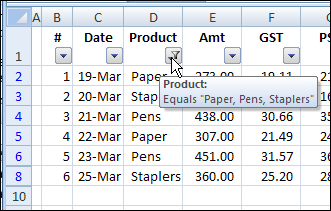________________